# Free Cash Flow (FCF) Yield

Understand the Concept of Unlevered and Levered FCF Yield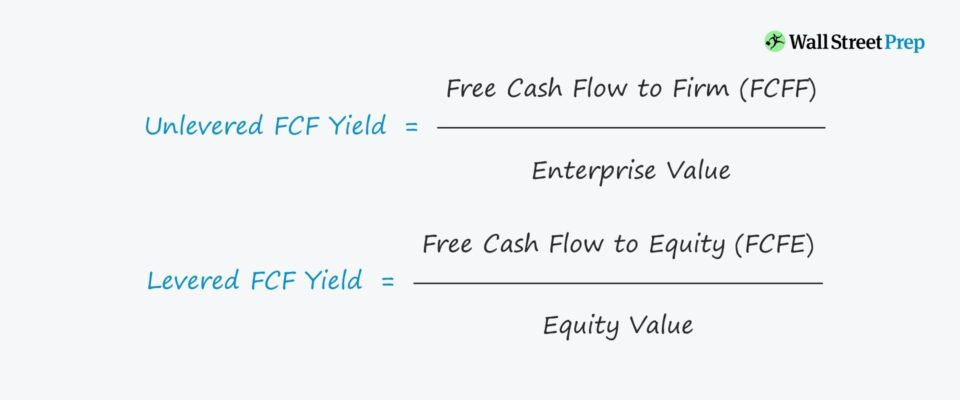• What is the meaning of free cash flow yield?
• Why is the FCF yield an important metric to track?
• How are the unlevered and levered free cash flow yields different?
• When could the unlevered or levered FCF yield be equivalent?

## Free Cash Flow (FCF) Yield Definition

The FCF yield metric matters because companies that generate more cash flow than they spend are less reliant on the capital markets for external financing.

Cash-flow generative companies are self-sufficient in being able to fund their growth plans themselves – and are thus worth more and valued at higher multiples by the market.

Based on whether an unlevered or levered cash flow metric is used, the free cash flow yield denotes how much cash flow that the represented investor group(s) are collectively entitled to.

• If the cash flow metric used as the numerator is “unlevered” free cash flow, the corresponding valuation metric in the denominator is enterprise value (TEV).
• On the other hand, if the cash flow metric is “levered” free cash flow, then the matching valuation metric would be the equity value.

## Unlevered vs Levered FCF Difference

In the two preceding posts, we discussed the formulas and steps to calculate:

To review the main differences between the two FCF types, reference the table below.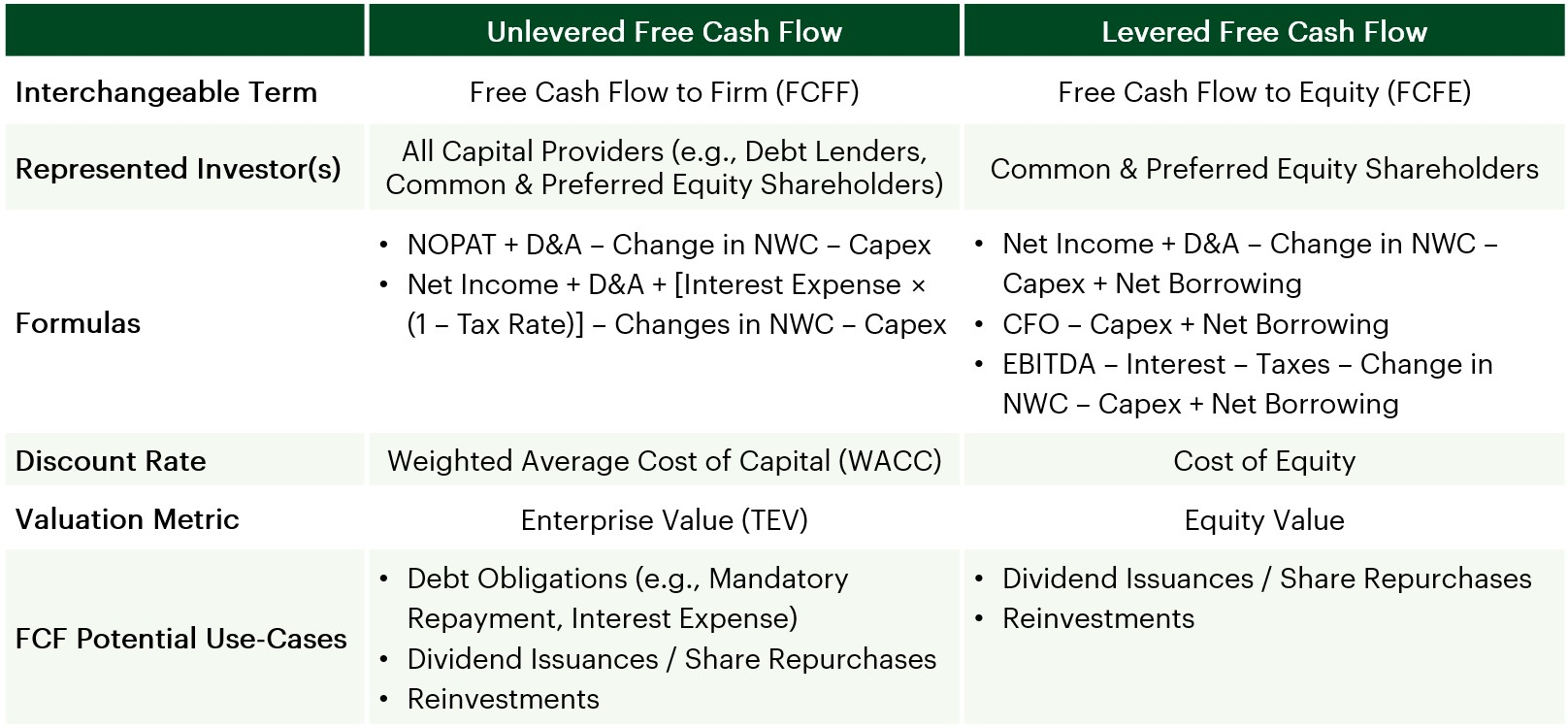## Unlevered Free Cash Flow (FCF) Yield Formula

Here, we will be discussing the formulas for calculating the FCF yield – or more specifically, the difference between the unlevered and levered FCF yield. FCF yield metrics are similar to valuation multiples in that the cash flow metric (numerator) is standardized to show a per-unit basis of the valuation (denominator).

###### Unlevered FCF Yield Formula
• Unlevered FCF Yield = Free Cash Flow to Firm / Enterprise Value

By standardizing in this way, the yields can be benchmarked against comparable companies (of different magnitudes of FCF), as well as to the company’s historical performance.

Otherwise, the FCF amount by itself would not provide much insight into the positive (or lackluster) progress of the company or how its recent trajectory lines up against its industry peers.

Another similarity to valuation multiples is that unlevered metrics are more widely used. Therefore, the unlevered FCF yield tends to be used for a broader range of purposes, while the levered FCF yield is more often tracked by private equity firms as well as investors in the public markets.

The unlevered FCF yield depicts the overall performance of the company on an operational level, and it can show the amount of remaining cash that could be put to use in order to benefit all providers of capital (debt and equity).

For instance, the cash could be used to pay down mandatory debt obligations, meet interest expense payments, issue a dividend to common or preferred equity holders, repurchase shares, or reinvest into the business.

We use the enterprise value as the corresponding valuation metric (denominator), which also represents the entire value of the company to all capital providers.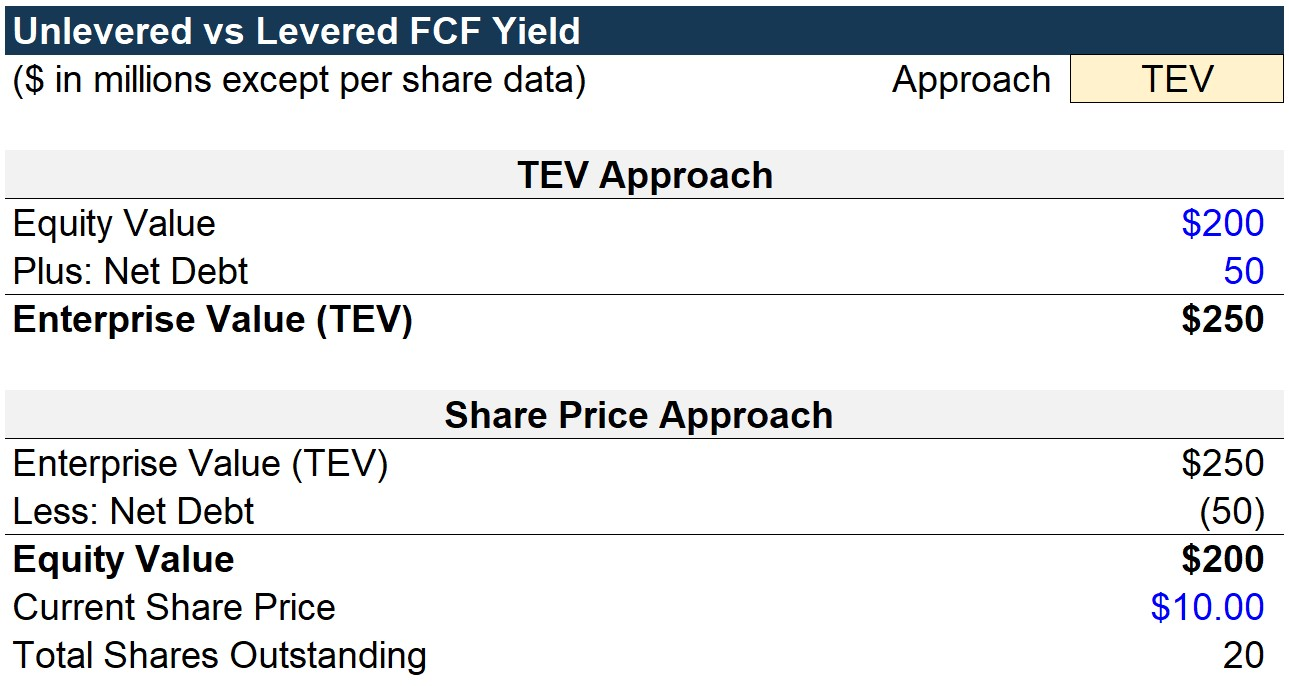## Levered Free Cash Flow (FCF) Yield Formula

In contrast, the formula for the levered free cash flow yield is the levered free cash flow divided by the equity value. Since the free cash flow to equity pertains only to equity holders, we must use the equity value in the denominator to match the represented stakeholders.

In short, the levered FCF yield tells equity holders the amount of residual free cash flow allocatable to each unit of equity value.

###### Levered FCF Yield Formula
• Levered FCF Yield = Free Cash Flow to Equity / Equity Value

Alternatively, the levered FCF yield can be calculated as the free cash flow on a per-share basis divided by the current share price.

The formula shown below is just a derivation of the formula above, as the only difference is that both the numerator and denominator were divided by the total number of shares outstanding.

###### Levered FCF Yield Formula
• Levered FCF Yield = Free Cash Flow Per Share / Current Share Price

Comparable to the dividend yield, the levered FCF yield can gauge the returns to equity holders relative to the share price of the company. The main shortcoming of the dividend yield metric, however, is that not all companies issue dividends.

As a result, the levered FCF yield tends to be more relevant and broadly used.

There is no single levered FCF yield that investors or operators target since the returns will vary by industry.

In addition, the levered FCF yield is impacted by several other factors such as the financing mix (debt-equity ratio) and required amortization of debt.

That being said, the levered FCF yield is usually viewed as more of an internal measure (i.e., to compare against the company’s own past performance and for investors to gauge their cash flow value per unit of equity) rather than a comparison metric against other competitors.

As an example, the levered FCF of a company relative to its industry peers could be on the lower end due to the presence of debt on its balance sheet and/or having unfavorable lending terms.

Generally speaking, the higher the levered FCF yield, the better, since this implies the company is generating more cash that could be used to benefit equity shareholders (e.g., dividends, buybacks) and reinvest into the growth of the business.

What matters more than the absolute metric itself is how the FCF yield compares on a year-over-year (YoY) basis and for the Company to understand and evaluate why (and if) the yield changed. From the perspective of a private equity investor, if the levered FCF yield increases over time, this is usually a positive sign that signifies downside protection and the ongoing ability of the Company to meet its debt obligations.

## Free Cash Flow (FCF) Yield – Excel Template

Before we get into our tutorial, fill out the form below to download the template: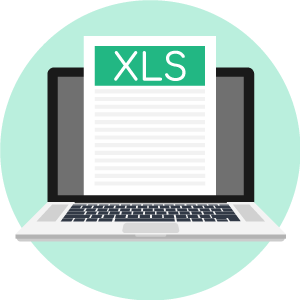Submitting ...

## Enterprise Value and Equity Value Calculation

To start, we are provided with information regarding the valuation of the company to calculate Total Enterprise Value (TEV) and equity value. Recall that TEV is the sum of the equity value and net debt.

To calculate TEV, we add equity value to net debt to arrive at TEV. The equity value of the company is \$200mm while the net debt is \$50mm, which are added together to arrive at a TEV of \$250mm.

To calculate equity value, we start with TEV and then subtract the net debt to get to equity value. We subtract \$50mm of net debt from the \$250mm TEV to arrive at \$200mm.

We are also given that the share price is \$10.00. Therefore, we can calculate the share count by dividing the \$200mm of equity value by the \$10.00 share price, which comes out to 20mm shares.## Unlevered FCF Calculation

We now analyze the financials given the operating assumptions provided at the bottom.

Since revenue is \$100mm and the EBITDA margin assumption is 40%, EBITDA comes out to \$40mm. We then subtract the \$10mm in depreciation and amortization (D&A) for an EBIT of \$30mm.

This figure will be tax-effected by the 30% tax rate, which will be a \$9mm reduction to get \$21mm in net operating profit after taxes (NOPAT).

Because interest was not subtracted from EBIT before applying the tax, the impact of interest had already been removed from the equation.

With NOPAT calculated, we can begin working our way towards free cash flow to firm (FCFF), or unlevered FCF.

As D&A is a non-cash expense, we will add back the \$10mm from earlier, which we originally subtracted because D&A is tax-deductible. But unlike interest, D&A is a core, operating line item that impacts all stakeholders.

Once we subtract the re-investment needs of the company, the \$5mm in CapEx and \$3mm in the change in net working capital (NWC), we arrive at the FCFF of \$23mm.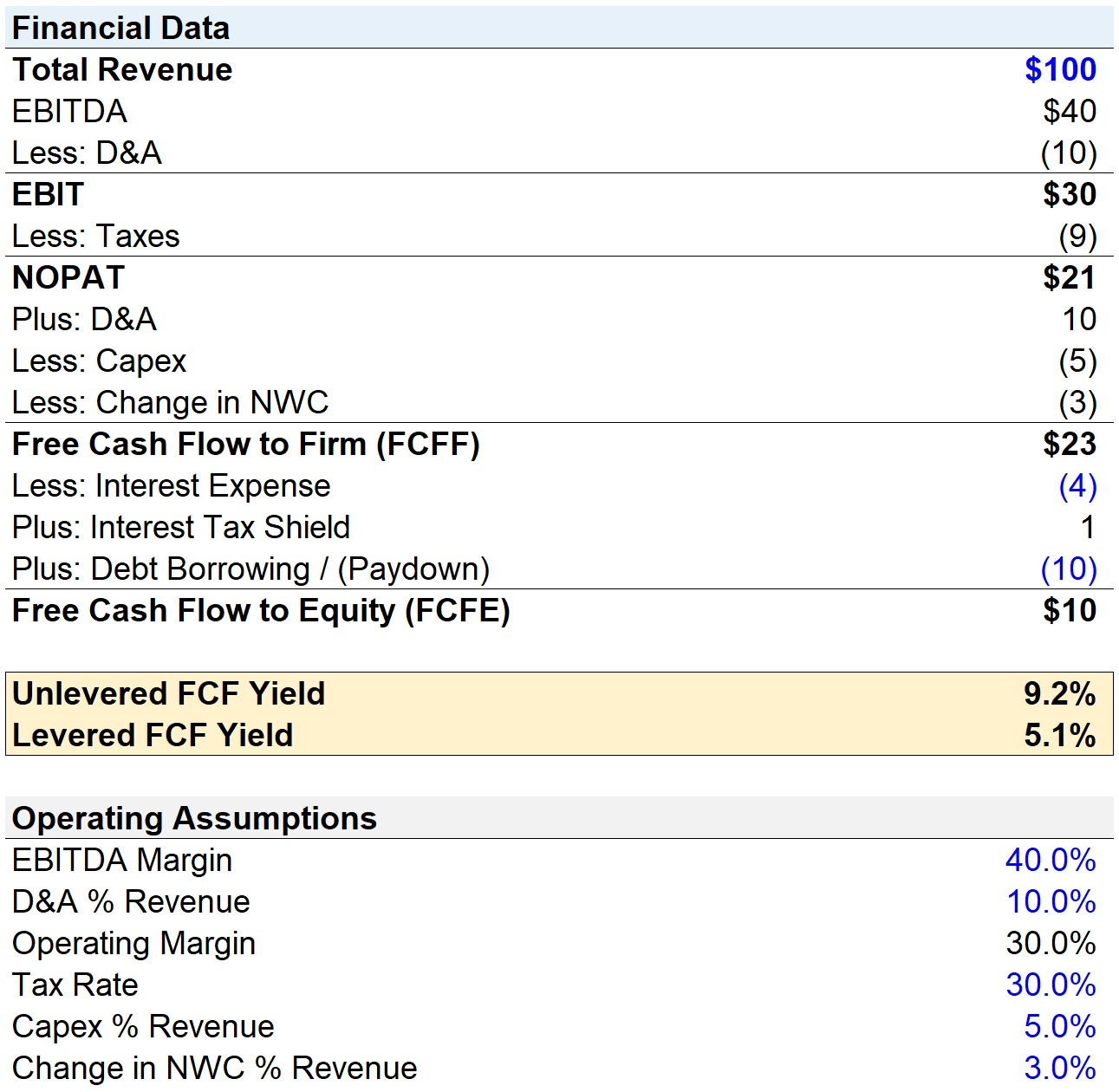## Levered FCF Calculation

At this point, we must now calculate levered FCF from unlevered FCF. Intuitively, the expenses that impact non-equity holders must be accounted for in this section, so that we are left with only the residual cash flows for equity.

The process is fairly straightforward and we will deduct three debt-related items:

1. Interest Expense: Periodic payments made to debt providers throughout the lending period, typically based on the outstanding debt principal (i.e., the cost of borrowing)
2. “Tax Shield” of Interest: Interest is tax-deductible and reduces the taxable income (or earnings before taxes, EBT)
3. Mandatory Debt Paydown: Required principal repayments back to the original provider per the lending agreement

So the first debt item is the \$4mm in interest expense, which is a hard-coded assumption here.

Then, the adjusted interest amount could be calculated by multiplying the interest expense by (1 – tax rate).

By entering the \$4mm interest assumption and 30% tax rate into the formula, we get \$2.8mm as the tax-adjusted interest. Here, we have explicitly broken out the tax shield, which is the tax savings associated with interest.

Note that the interest tax shield is shown as a cash inflow since the tax savings are beneficial to all capital providers.

The final debt-related obligation is the mandatory repayment of \$10mm. After summing up the FCFF with the three-line items mentioned above, the levered FCF comes out to \$10mm.

## FCF Yield Calculation – Unlevered vs Levered

In our final section, we can calculate the unlevered and levered FCF yields.

For the unlevered FCF yield, we have an “IF” function saying that if the approach toggle selected is on “TEV”, then the FCFF of \$23mm will be divided by the TEV of \$250mm.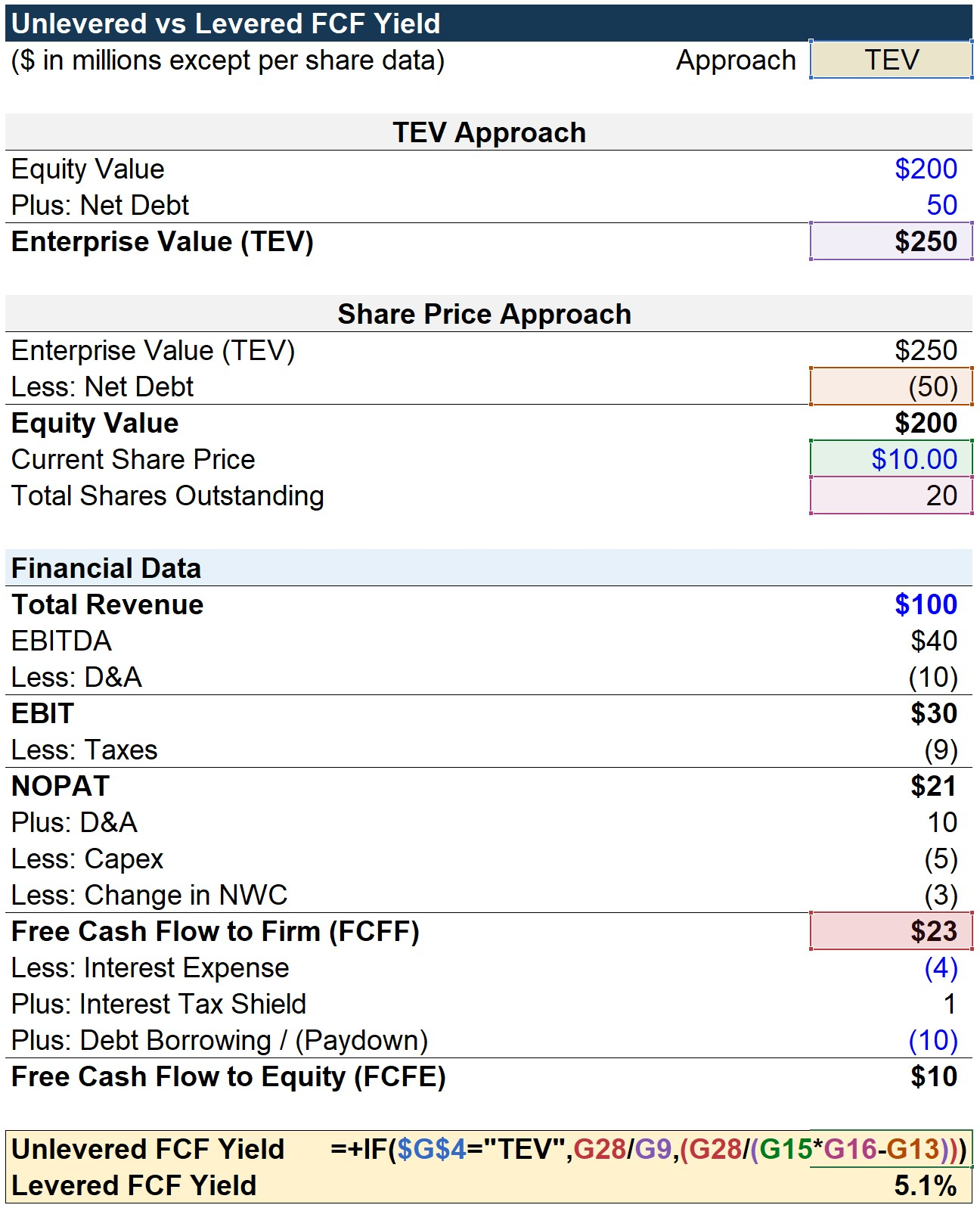If the toggle is set on “Share Price” instead, then the \$10.00 share price is multiplied by the 20mm in shares outstanding to get the equity value.

Recall, the valuation metric that corresponds to FCFF is the enterprise value, so we add the \$50mm in net debt to arrive at the TEV in the denominator.

In either case, the unlevered FCF yield comes out to 9.2%. For the “Share Price” toggle approach, the reason we did not just link to the equity value and add net debt is to show an alternative approach where the formula is more dynamic, where we can adjust the calculation as under different assumptions for the share price.

The levered FCF yield formula also contains an “IF” function. In the first portion, if the approach toggle is set to “TEV”, then the FCFE of \$10mm is divided by the \$200mm in equity value.

Otherwise, if the toggle is on “Share Price”, the FCFE is divided by the current share price multiplied by the total shares outstanding. In this case, there is no need to add back net debt, since we want the denominator to be the equity value.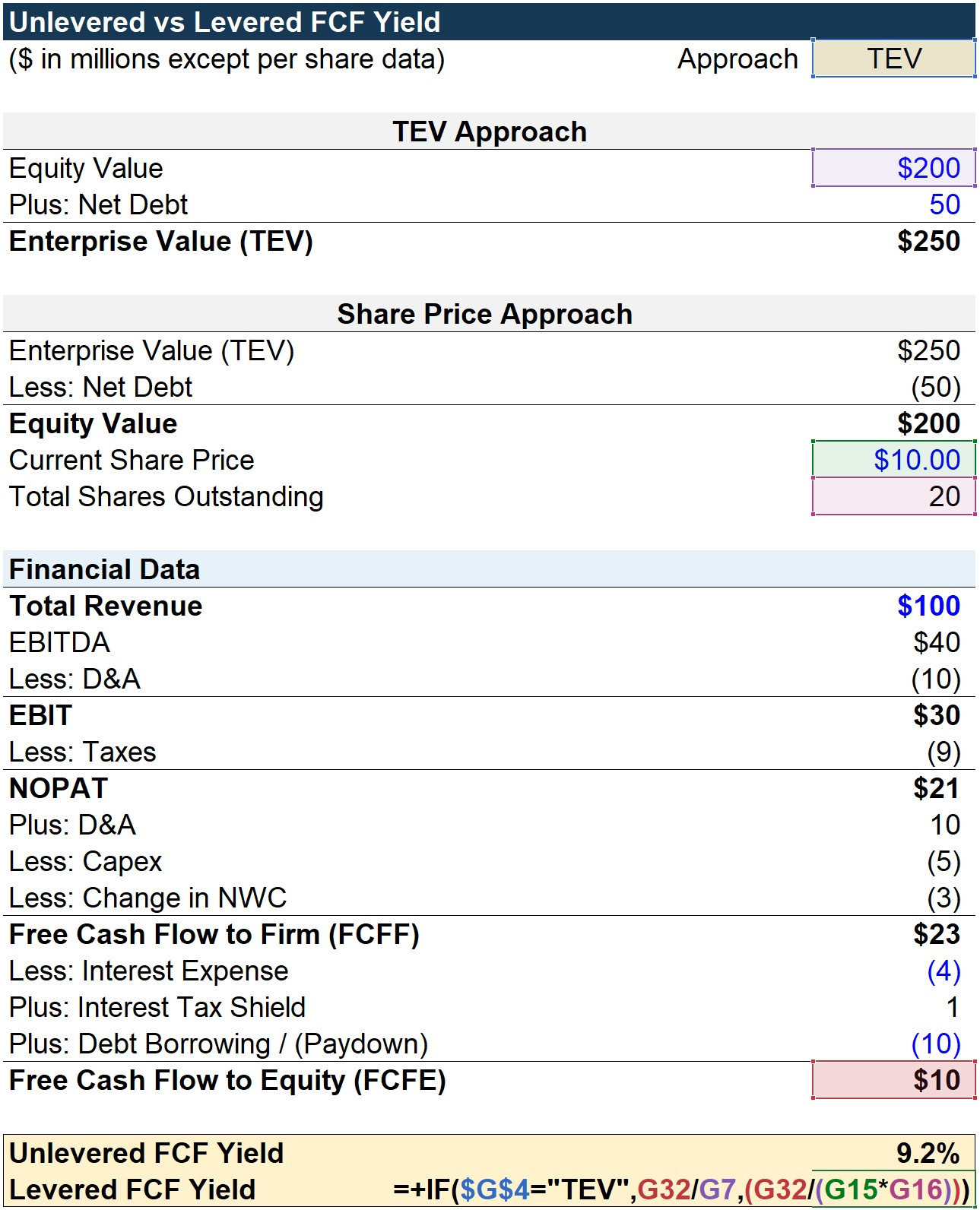The completed model output is shown below. The levered FCF yield comes out to 5.1%, which is roughly 4.1% less than the unlevered FCF yield of 9.2% due to the debt obligations of the company.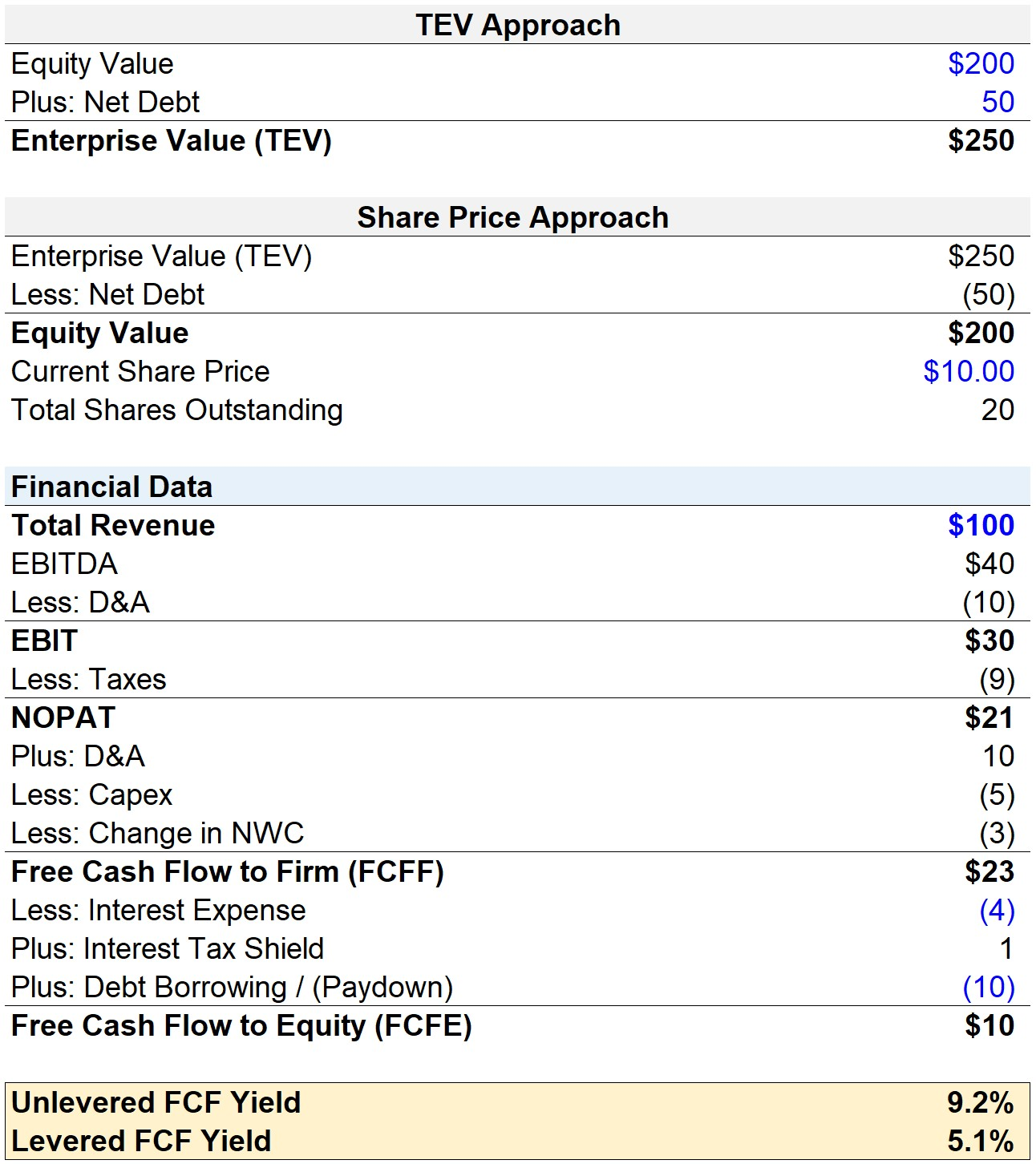If all debt-related items were removed from our model, then the unlevered and levered FCF yields would both come out to 11.5%.

It should be intuitive that the unlevered and levered FCF yields are equivalent for all-equity companies that hold no debt on their balance sheet since the only difference between the two arises from the effects of leverage (debt).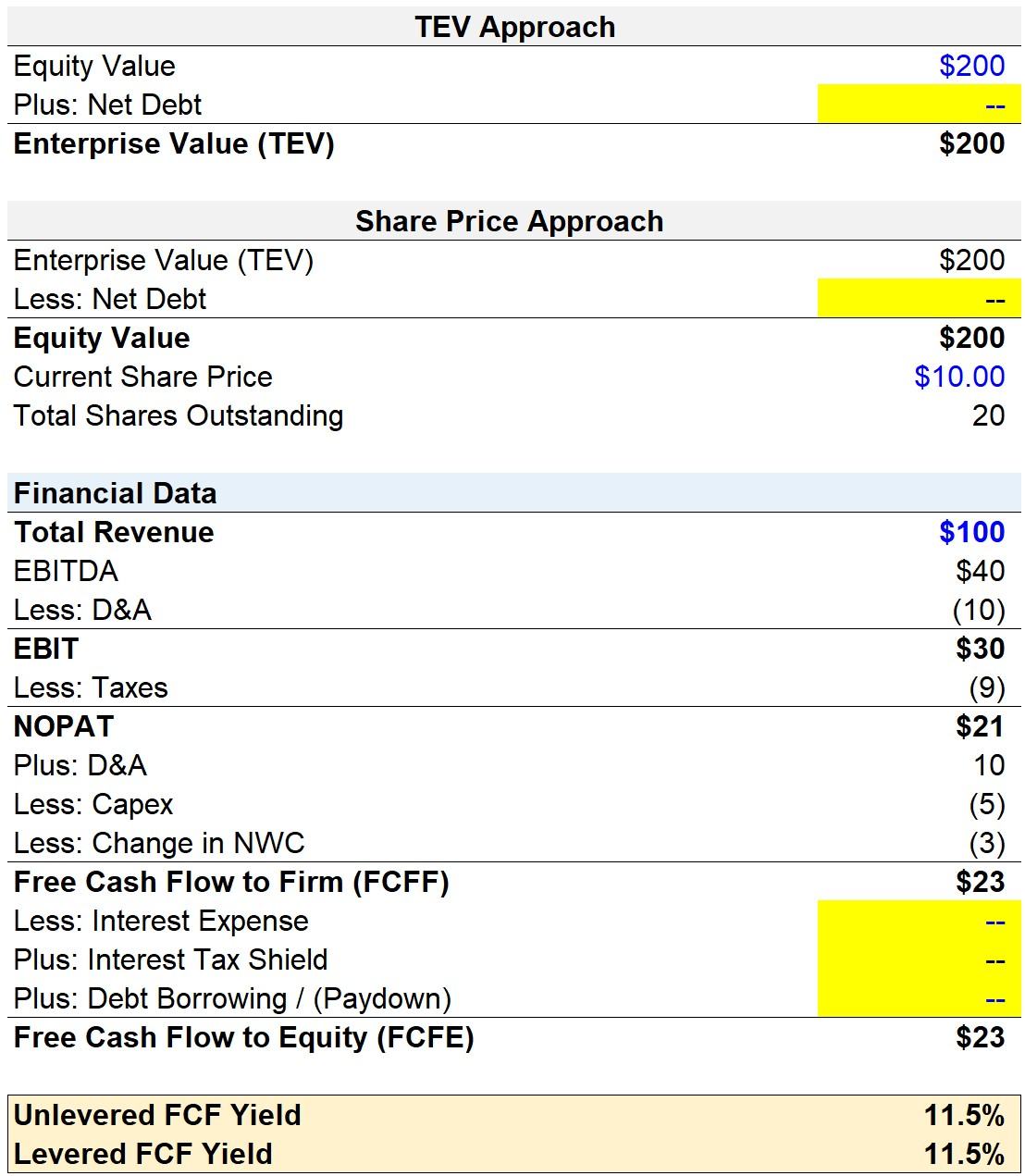Step-by-Step Online Course

#### Everything You Need To Master Financial Modeling

Enroll in The Premium Package: Learn Financial Statement Modeling, DCF, M&A, LBO and Comps. The same training program used at top investment banks.Inline FeedbacksLearn Financial Modeling Online

Everything you need to master financial and valuation modeling: 3-Statement Modeling, DCF, Comps, M&A and LBO.

X

The Wall Street Prep Quicklesson Series

7 Free Financial Modeling Lessons

Get instant access to video lessons taught by experienced investment bankers. Learn financial statement modeling, DCF, M&A, LBO, Comps and Excel shortcuts.